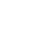# Kotlin Algorithm Challenge #3Alex Woods

January 20, 2020

The following problem is the Reverse Integer problem on LeetCode.

# Problem

Given a 32-bit signed integer, reverse its digits.

``````fun reverse(x: Int): Int {
// TODO
}
``````

# Solution

This problem is not too difficult (i.e. this is absolutely a fair interview question!). The approach we’re going to take is **breaking down the number by multiples of 10, and using the broken off piece to construct the reversed number**.

There might be a temptation to convert the value to a string, reverse it, then convert it back again. Don’t do that, it’s completely against the spirit of the problem. I also trust math more than I trust type conversion, if code like this were to ever make it into production.

``````fun reverse(x: Int): Int {
var int = x
var sum = 0

while (int != 0) {
val digit = (int % 10)

val newSum = 10*sum.toLong() + digit.toLong()
check(newSum > Integer.MIN_VALUE && newSum < Integer.MAX_VALUE) {
"Integer overflowed, input too large"
}

sum = newSum.toInt()
int /= 10
}

return sum
}
``````

You can see the progression of our `sum` variable in the following example.

``````1234
10*0 + 4 = 4
10*4 + 3 = 43
10*43 + 2 = 432
10*432 + 1 = 4321
``````

# Writing Good Kotlin Code

But how does idiomatic Kotlin code fit into this problem?

## Pure Functions

A pure function is a function that doesn’t mutate it’s input, and it has no side effects. Because of these two things, when a pure function is run multiple times with the same input, it gives the same result.

It’s not obvious in the above code, but Kotlin is making me go out of my way to write a pure function. Try the following:

``````fun foo(x: Int) {
x = 4  // an error pops up: "Val cannot be reassigned"
}
``````

In a similar vein, collections are immutable by default.

``````fun foo(list: List<Int>) {
list.add(4) // nope! the compiler won't allow it!
}
``````

The above isn’t allowed, because the `List` interface extends the `Collection` interface, which doesn’t contain an `add` method. If you want an add method, you have to declare `MutableList`, which extends `MutableCollection`. And putting `MutableList` as a type of a function input parameter should set off a red flag in your head.

## Check

The `check` function checks some condition. If it is `false`, it throws an `IllegalStateException`. We can use it to make sure people are only calling our function at the right time.

Similar to `check` is the function `require`. We pass it a condition, and if `false`, it throws an `IllegalArgumentException`. So it is used for validating input. e.g.

``````fun poundsToKilograms(weightInPounds: Double) {
require(weightInPounds >= 0) {
"There's no such thing as a negative weight."
}

return weightInPounds / 2.2
}
``````

## Augmented Assignment Operators

The slightly funny looking operator `/=` means divide and then assign. That line is equivalent to the following.

``````int = int / 10
``````

Want to know when I write a new article?

Get new posts in your inbox